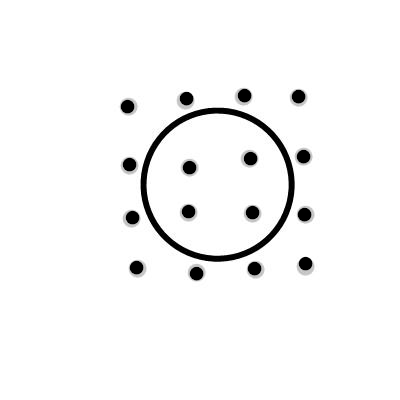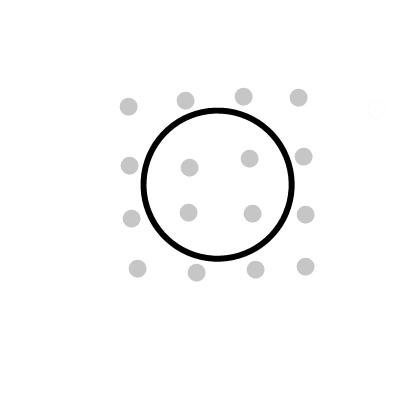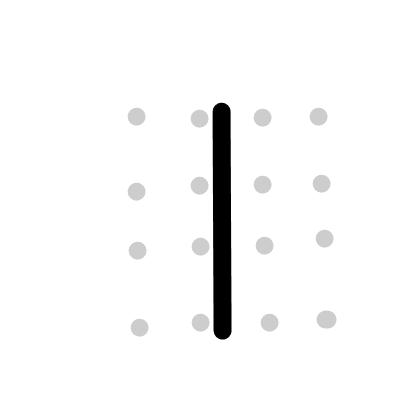# Find the size and direction of the induced current of the given three loops from the figures A,...

## Question:

Find the size and direction of the induced current of the given three loops from the figures A, B, C where the diameter of three loops are 13-cm and the loop's resistance of the three loops are 0.24 {eq}\Omega {/eq} using the condition below the figure.

Figure AFigure B.Figure C.## Electromagnetic Induction

The phenomenon of induction of emf in a closed conducting loop due to change in magnetic flux linked with the loop is known as electromagnetic induction.

Magnitude of induced emf is equal to the rate of change in flux and induced current is directed in a way to oppose the change which causes it.

Induced emf is equal to rate of change in magnetic flux .

Thus

{eq}\varepsilon = \dfrac{d \phi}{dt} {/eq}

{eq}\varepsilon = \dfrac{d...

Become a Study.com member to unlock this answer! Create your account